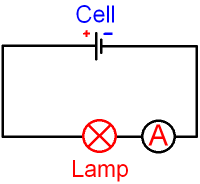gcsescience.com                                       4                                       gcsescience.com

Electricity

What is Electric Current?

Current is the "rate of flow of coulombs" in
an electric circuit. A coulomb is a unit of charge,
so an electric
current is a flow of charge.
Charge is given the symbol Q.

Current is given the symbol I.
This is the capital letter I, not a number 1.
Current is measured in amps.

What is an
Amp?

1 amp = 1 coulomb per second.

The word "per" means "divided by",
so  current = charge ÷ time.    I = Q ÷ t.

We can calculate the current if we know
the charge and the time.

This equation can be rearranged to give
charge = current x time.    Q = I x t.

Current can also be calculated using
I = P ÷ V   and   I = V ÷ R

See all equations for electricity.

How is Current measured?

Current is measured using an ammeter.The circuit diagram above shows how to measure
the current flowing through a lamp. The circuit symbol
for an ammeter is a circle with the letter A inside. An
ammeter is always connected in series with a component.

If the ammeter reads 1 amp,
then the current (I) = 1 amp at that point in the circuit.
I = 1 amp = 1 coulomb per second.

If the ammeter reads 6 amps,
then I = 6 amps = 6 coulombs per second.

Current has a heating effect
and thick wire must be used to carry a large current.

gcsescience.com      Physics Quiz      Index      Electricity Quiz      gcsescience.com